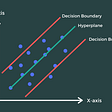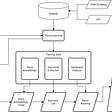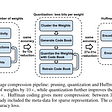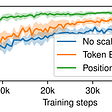# Baby steps in Neural Machine Translation Part 1 (Encoder) — Human is solving the challenge given by the God

• Walk your through the concept of current machine translation system — Attention is All you Need.
• Step-by-step, guide you through the codes with brief explanation.

Once upon a time, all humans speak the same language. They plan to build a tower (Tower of Babel) that can reach the heaven but this has angered the God. While humans were building up the tower, the God was building the language barrier. Suddenly, humans cannot understand each other and stop building the city.

However, the story continues with new chapter. Humans never stop trying to understand each other. We created dictionaries, language rules, statistical machine translation and now, we reached another milestone — Neural Machine Translation (NMT).

We will start this blog with high level concept of translation model. Then we will walk your through the codes.

In NMT, we will look at all the words in source sentence and think of the appropriate translation. Then, we start to write down the first translated word by taking into consideration of the tense info, part of speech, sentence structure, etc. Next, we will write down the second word by looking at the source sentence and the translated word written down previously. Repeatably, we will write down translated word one by one until we feel that the translation is complete. This is the high level concept of machine translation system. But how is this concept implemented in Machine?

This is actually a seq-to-seq deep learning model which consist of encoder and decoder. The encoder is used to learn the language information from source sentences while the decoder is used to learn the language information from target sentences during training. During prediction, the decoder will take in information from encoder and predict one word by one word to form a complete translated sentence. We will start explaining from the easiest part of NMT — Encoder.

In Encoder part, we will feed in source sentence which has gone through pre-processing steps such as tokenization and indexing. These 2 steps are basically splitting sentence into words and assigning each word with a unique number also known as word id.

`tokenizer_en = tfds.features.text.SubwordTextEncoder.build_from_corpus(    (en.numpy() for pt, en in train_examples), target_vocab_size=2**13)tokenizer_pt = tfds.features.text.SubwordTextEncoder.build_from_corpus(    (pt.numpy() for pt, en in train_examples), target_vocab_size=2**13)`

There are several ways to tokenize a sentence. The easiest way to tokenize a sentence is by using an empty space “ ”. But there are some languages don’t use space in their sentences such as Chinese and Thai. So, byte-pair encoding (BPE) is introduced to tokenize a sentence. The basic concept is that we tokenize a sentence according to the common characters.

`sentence 1 : "I go to school"sentence 2 : "I go to mall"BPE tokens:token 1 : I go totoken 2 : schooltoken 3 : mallempty space tokens:token 1 : Itoken 2 : gotoken 3 : totoken 4 : schooltoken 5 : mall`

BPE tokenization method reduces the number of tokens and this method alos solves another common translation problem which is known as out-of-vocabulary problem (OOV) because BPE can generate tokens with only one character “a, b, c…”. The next step would be indexing. Indexing is the process of converting tokenized word into number.

`Using BPE token:sentence 1 : "I go to school" => [1, 2]sentence 2 : "I go to mall" => [1, 3]`

In the example above, sentence 1 is indexed into a vector of [1, 2]. This step is completed with the tensorflow build-in encode function.

`sample_string = 'Transformer is awesome.'tokenized_string = tokenizer_en.encode(sample_string)print('Tokenized string is {}'.format(tokenized_string))original_string = tokenizer_en.decode(tokenized_string)print('detokenized string is {}'.format(original_string))assert original_string == sample_stringTokenized string is [7874, 3305, 5284, 5397, 334, 15, 1940, 292, 2422, 7836]detokenized string is Transformer is awesome.`

Now, we have the indexed source sentence and this sentence is ready to be passed to the embedding layer in the Encoder part. Let’s give some dimension to the indexed source sentence so that we know the transformation of indexed source sentence when it flows through the encoder part. We assume that we parallel process 64 sentences together and each sentence has 62 words. If the sentences does not have 62 words, we will append 0 to the indexed sentences. So, the dimension of the indexed sentences would be (64, 62).

`sentence 1 : "I go to school" => [1, 2] => [1,2,0,0,0,0,0,0,0,0,0,0,0,0,0,0,0,0,0,0,0,0,0,0,0,0...]`

The embedding layer in the Encoder part creates a new dimension to the indexed sentences. We will call the embedding layer output as source embedded tensors (64, 62, 512). Actually, we can think that the embedded tensors carries the language information of each word. Every word has 512 features. The features could be tenses, part of speech and etc. Yet, the 512 features do not contain the word position information. The word position information is elegantly generated using Sin and Cos function and added with source embedded tensors.

• Position embedding
`def get_angles(pos, i, d_model):  angle_rates = 1 / np.power(10000, (2 * (i//2)) / np.float32(d_model))  return pos * angle_ratesdef positional_encoding(position, d_model):  angle_rads = get_angles(np.arange(position)[:, np.newaxis],                          np.arange(d_model)[np.newaxis, :],                          d_model)    # apply sin to even indices in the array; 2i  angle_rads[:, 0::2] = np.sin(angle_rads[:, 0::2])    # apply cos to odd indices in the array; 2i+1  angle_rads[:, 1::2] = np.cos(angle_rads[:, 1::2])      pos_encoding = angle_rads[np.newaxis, ...]      return tf.cast(pos_encoding, dtype=tf.float32)`

When we have 62 words in a sentence, we will create 62 position embedding. The even positions (0,2,3,4,6,8,10..62) embedding are generated using Sin function while the odd positions (1,3,5,7,9,11…25) embedding are generated using Cos function. Basically, every word has a unique position embedding. Now, we have generated positions embedding (1, 62, 512) and from the encoder embedding layer, we have source embedded tensors (64, 62, 512). By adding generated positions embedding (1, 62, 512) and source embedded tensors (64, 62, 512), we have source embedding with position tensors (64, 62, 512). After that, these tensors will flow to multi-head attention layer which composes of split head function and scaled dot-product attention module.

• Scaled Dot-Product Attention

This scaled Dot-Product Attention module is the gist of the entire machine translation system. Therefore, we will explore this module step-by-step with codes and math explanation. Let’s us start with the simple math equation. In this equation, we see three input tensors Q, K, and V. In the encoder part, Q, K, V take in source embedding with position tensors (64, 62, 512). Q, K and V have the same dimension which is (64, 62, 512).

When we go deeper into Q* Transpose(K), we know that this is actually (64, 62, 512) * (64, 512, 62) and result in (64, 62, 62). What does this mean?

This mean we are representing word 1 (w1) from the perspective of other words. Alternatively, we could say that we are find the similarity of word 1 with other words in latent space. Let’s look into the highlighted matrix output. w1 has a new vector containing the information from w2. Let’s call the matrix output as word-from-words tensors (64, 62, 62). Then, we perform softmax operation on the word-from-words tensors (64, 62, 62). This process selects the most important feature from the word-from-words tensors (64, 62, 62) to be emphasized/highlighted for the prediction of current targeted word. The highlighted features (64, 62, 62) do not contain the language information such as tenses and part of speech as mentioned earlier. The last step is to get the language information of the emphasized/highlighted features by multiplying with embedded source tensors V (64, 62, 512). The output dimensions of this multiplication would be (64, 62, 62) * (64, 62, 512) = (64, 62, 512). At this moment, we have 62 words with highlighted features from the perspectives of other words.

For the square root value, basically it is just to reduce the magnitude of Q*Transpose(K) and increase the training speed because the square root value is applied to all matrix output. We can treat the square root value as constant.

`def scaled_dot_product_attention(q, k, v, mask):    """    q = k = v = inputs     args:     q : query shape == (batch, heads, seq_len_q, depth) eg. (64, 8, 2, 512)    k : key shape == (batch, heads, seq_len_k, depth) eg. (64, 8, 2, 512)    v : value shape == (batch, heads, seq_len_v, depth_v) eg. (64, 8, 2, 512)    mask : float tensor with shape broadcastable to (batch, seq_len_q, seq_len_k). Defaults to None.        returns:        output, attention_weights    """        matmul_qk = tf.matmul(q, k, transpose_b=True) # (64, 8, 2, 512) * (64, 8, 512, 2) = (64, 8, 2, 2)    # q and k are the same input words     # matmul qk can produce new vectors for each for word where each word contains the information of other words        # scale matmul_qk    dk = tf.cast(tf.shape(k)[-1], tf.float32)    scaled_attention_logits = matmul_qk / tf.math.sqrt(dk) # (64, 8, 2, 2)        # add the mask to the scaled tensor    if mask is not None:        print("before mask scaled_attention_logits : ", scaled_attention_logits.shape)        print("mask ：", mask.shape)        scaled_attention_logits += (mask * -1e9)        print("after mask scaled_attention_logits : ", scaled_attention_logits.shape)            # softmax is normalized on the last axis (seq_len_k) so that the scores add up to 1     # and attent the important feature            attention_weights = tf.nn.softmax(scaled_attention_logits, axis=-1) # (batch, heads, seq_len_q, seq_len_k)            # select the important words to attend    output = tf.matmul(attention_weights, v) # (64, 8, 2, 2) * (64, 8, 2, 512) = (64, 8, 2, 512)            return output, attention_weights`

With the solid basic on scaled dot-product attention, we will go into multi-head attention which is the upgrade of dot-production attention that can make the training and prediction faster. As described earlier, scaled dot-product attention module takes in source embedded tensors (64, 62, 512) and the output is the words with highlighted features from the perspectives of other words (64, 62, 512).

For multi-head attention, we split the dimension of the source embedded tensors (64, 62, 512) into (64, 8, 62, 64). Intuitively, we split the 62 words with 512 language features into 8 groups of 62 words with 64 language features. Then, the similar processes in scaled dot-product attention module are performed on the 8 groups word features (64, 8, 62, 64).

• softmax(Q*Transpose(K)) = softmax( (64, 8, 62, 64) * (64, 8, 64, 62) ) = softmax((64, 8, 62, 62)) = (64, 8, 62, 62)
• softmax(Q*Transpose(K)) * v = (64, 8, 62, 62) * (64, 8, 62, 64) = (64, 8, 62, 64)

Since we split the 512 features into 8 groups of 64 features, we have to concatenate back 8 groups to form 512 features (64, 62, 512). And since we process the 8 groups concurrently, the training speed and prediction speed increases significantly.

`class MultiHeadAttention(tf.keras.layers.Layer):    def __init__(self, d_model, num_heads):        super(MultiHeadAttention, self).__init__()        self.num_heads = num_heads        self.d_model = d_model                # check whether it can be split        assert d_model % self.num_heads == 0                self.depth = d_model // self.num_heads                self.wq = tf.keras.layers.Dense(d_model)        self.wk = tf.keras.layers.Dense(d_model)        self.wv = tf.keras.layers.Dense(d_model)                self.dense = tf.keras.layers.Dense(d_model)            def split_heads(self, x, batch_size):        """        x shape == (batch_size, words_in_a_sent, word_features)        Split the words sequence into (num_heads, depth)        Transpose the result such that the shape is (batch_size, num_heads, seq_len, depth)        """                    # keep the feature dim, and batch size         # change the words_in a sent to group of words according to the heads number        x = tf.reshape(x, (batch_size, -1, self.num_heads, self.depth))                    return tf.transpose(x, perm=[0,2,1,3])            def call(self, v, k, q, mask):                batch_size = tf.shape(q)                    q = self.wq(q) # (batch_size, seq_len, d_model)        k = self.wk(k) # (batch_size, seq_len, d_model)        v = self.wv(v) # (batch_size, seq_len, d_model)        print(q.shape)                    q = self.split_heads(q, batch_size) # (batch_size, num_heads, seq_len_q, depth)        k = self.split_heads(k, batch_size) # (batch_size, num_heads, seq_len_k, depth)        v = self.split_heads(v, batch_size) # (batch_size, num_heads, seq_len_v, depth)                    # scaled_attention.shape == (batch_size, num_heads, seq_len_q, depth)        # attention_weights.shape == (batch_size, num_heads, seq_len_q, depth)                    scaled_attention, attention_weights = scaled_dot_product_attention(q, k, v, mask)                    # join multiple heads back by reducing one dimension. Have to revert back the head and seq before joining multiple heads        scaled_attention = tf.transpose(scaled_attention, perm=[0,2,1,3])        concat_attention = tf.reshape(scaled_attention, (batch_size, -1, self.d_model))                    output = self.dense(concat_attention) # (batch_size, seq_len_q, d_model)                    return output, attention_weights`

The last part of the Encoder is the Add & Norm Layer

In this module, we are actually normalizing the last 512 features using mean and standard deviation. x = (x-mean) / standard deviation. With the normalization, we are scaling a wide range of values into a smaller range of value which will help the update of weights faster. Let’s look at the example below:

`data =  [0, 5, 10, 1000] n = len(data)mean = sum(data)/nvariance = sum([((x - mean) ** 2) for x in data]) / nstddev = variance ** 0.5print("mean : ", mean, ", standard deviation : ",stddev)norm = [(i-mean)/stddev for i in data]print("ori value : ", data)print("norm value : ", norm)Output:mean :  253.75 , standard deviation :  430.8621444267296ori value :  [0, 5, 10, 1000]norm value :  [-0.588935471083493, -0.5773308312591877, -0.5657261914348825, 1.731992493777563]`

If the data is not normalized, we have the values range from 0 to 1000. After the data is normalized, the values range from -0.588935471083493 to 1.731992493777563.

`# standard normalization proving for LayerNormalization functiondata = tf.constant(np.arange(15).reshape(5, 3) * 5, dtype=tf.float32)print(data)layer = tf.keras.layers.LayerNormalization(axis=1, epsilon=1e-6)output = layer(data)print(output)data =  [0, 5, 10] n = len(data)mean = sum(data)/nvariance = sum([((x - mean) ** 2) for x in data]) / nstddev = variance ** 0.5print(mean, stddev)norm = (10-mean)/stddevprint("norm value : ", norm)`

With that, we finish the Encoder part. For the next blog, we will go through the explanation of decoder part. This is a very interesting part where we will looking into look-ahead mask and the usage of encoder output to train machine translation model. And I will show you the result from my translation model and where to get the data to train a customized translation model.

Here is the link to the decoder part:

https://medium.com/@alex_yeo/baby-steps-in-neural-machine-translation-part-2-decoder-human-is-solving-the-challenge-given-by-4d097c961e67

--

--

--

## More from Machine Growth

Angel is hiding in the details

## Reinforcement Learning Algorithms (Part 1)## Even our Machines are Racist## Neural Networks And It’s Use cases## Evaluating a Machine Learning Model## Sarus just released DP-XGBoost## Support Vector Regression Explained with Implementation in Python## Stock Recommendation System## Contextual v/s Non-Contextual Word Embedding Models For Hindi Named Entity Recognition## Breakdown and Utilization of a Convolutional Neural Network## Quantization in Deep Neural Networks## Compositional Generalization in Semantic Parsing (EMNLP 2021 paper notes)## Introducing Autoencoder